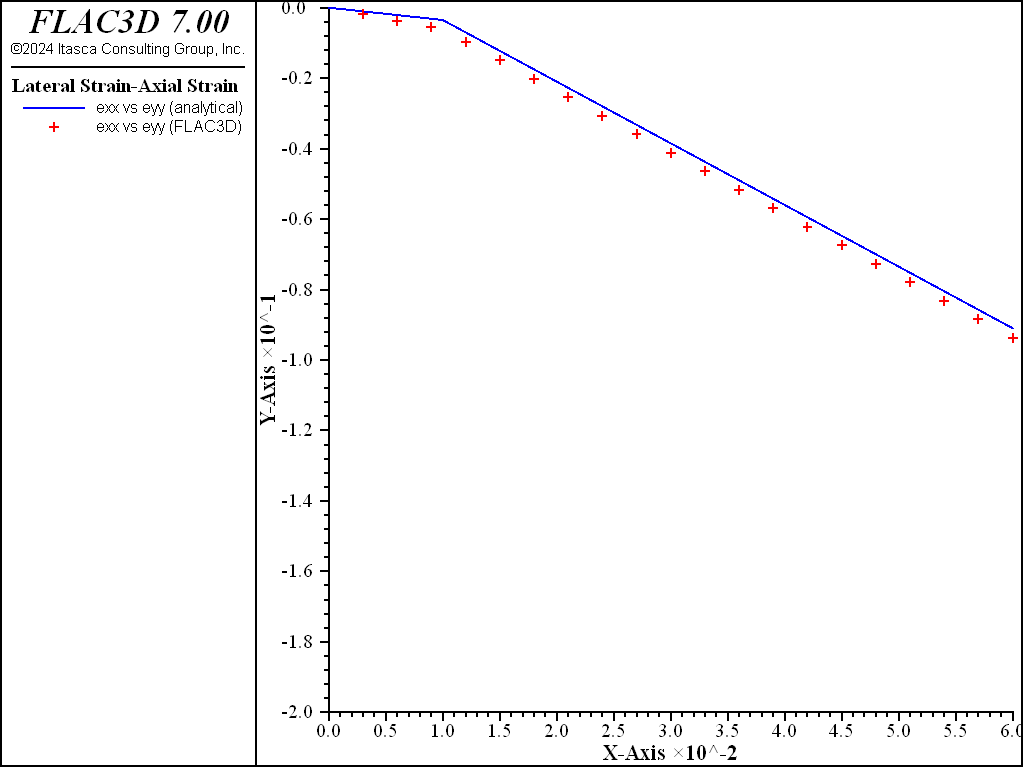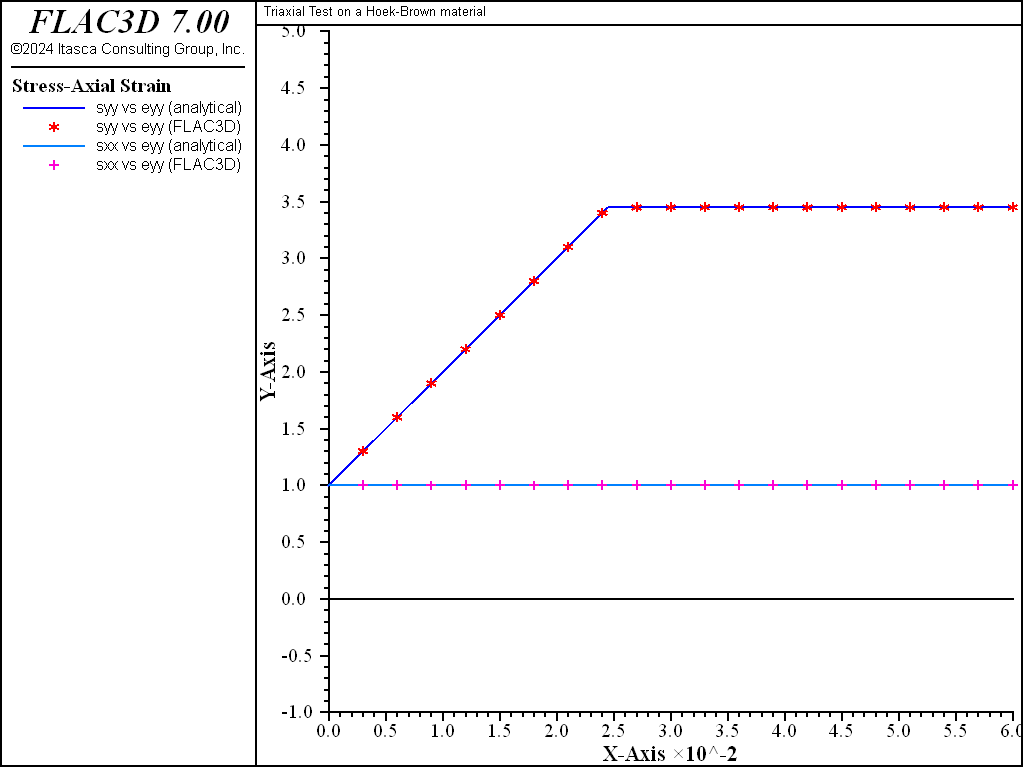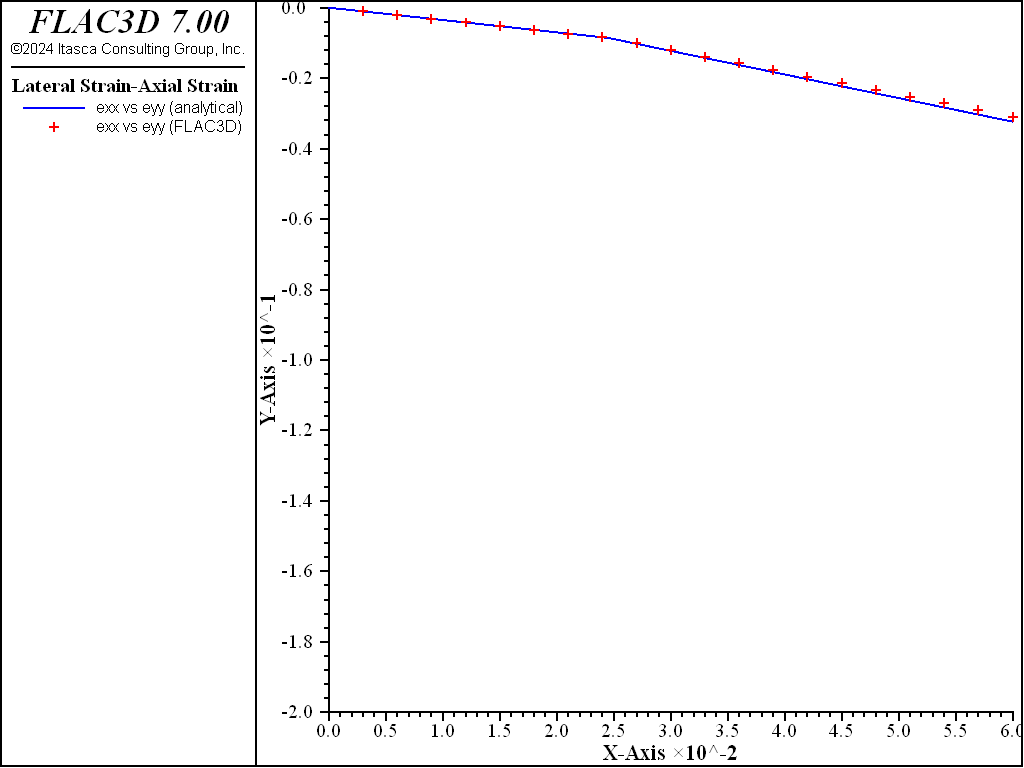FLAC3D Theory and Background • Constitutive Models

# Triaxial Compression Test with Hoek-Brown Model

Note

The project file for this example is available to be viewed/run in FLAC3D. The project’s main data file is shown at the end of this example.

The triaxial compression tests performed using the Hoek-Brown-PAC model are repeated for the Hoek-Brown model, with the same material properties:

 $$m_b$$ 5 $$s$$ 0.5 $$\sigma_{ci}$$ 1.0 MPa $$\sigma^{cv}_3$$ 1.5 MPa $$E$$ 100 MPa $$\nu$$ 0.35

at zero confining stress, $$\sigma_{3}/\sigma_{ci}$$ = 0, and

at high confining stress, $$\sigma_{3}/\sigma_{ci}$$ = 1.

For the zero confining stress case, we specify an associated flow rule; this is done with the command:

zone property flag-dilation = -1


For the higher confining stress case, we need to specify a dilation angle that is consistent with the limiting constant-volume stress, $$\sigma^{cv}_3$$ = 1.5, chosen for the triaxial compression test for the Hoek-Brow-PAC model. We linearly interpolate a value for dilation corresponding to the current confining stress level of $$\sigma_{3}$$ = 1, relative to a nonassociated zero dilation at $$\sigma^{cv}_3$$ = 1.5. The current dilation, $$\psi_c$$, is then taken to be a fraction of the current friction angle, $$\phi_c$$, using the linear interpolation:

${\psi_c \over \phi_c} = 1 - {\sigma_3 \over \sigma^{cv}_3} = 0.333$

The following commands are used to apply the modified Hoek-Brown model for this case:

zone property flag-dilation = 0.333


The FLAC3D results for the zero confining stress case are compared to the analytical solution (see Triaxial Compression Test with Hoek-Brown-PAC Model) in Figure 1 and Figure 2, and the results for the high confining stress case are compared in Figure 3 and Figure 4.

breakFigure 1: Triaxial compression test—stress versus axial strain ($${\sigma_3}/{\sigma_{ci}}$$ = 0).Figure 2: Triaxial compression test—lateral strain versus axial strain ($${\sigma_3}/{\sigma_{ci}}$$ = 0).Figure 3: Triaxial compression test—stress versus axial strain ($${\sigma_3}/{\sigma_{ci}}$$ = 1.0).Figure 4: Triaxial compression test—lateral strain versus axial strain ($${\sigma_3}/{\sigma_{ci}}$$ = 1.0).

Data File

TriaxialCompressionHoekBrown.dat

; Triaxial tests on a Modified Hoek-Brown material,
model new
model large-strain off
fish automatic-create off
model title "Triaxial Test on a Hoek-Brown material"
program call 'input_record'
[input]
zone create brick size 1 1 1
zone cmodel assign hoek-brown
zone property density = 1.0 shear=[shear]    bulk=[bulk]
zone property constant-sci=[sig_ci] constant-mb=[mb] ...
constant-s=[s] constant-a=[a]
[locptrs]
zone face apply velocity-y [y_vel] range position-y 1.0
zone face apply velocity-y [-y_vel] range position-y 0.0
fish history record_variables
history interval 1000
fish history eps_xx
fish history eps_yy
fish history eps_yy
fish history sig_xx
fish history sig_yy
fish history sig_zz
model save 'ini'
; unconfined
zone property flag-dilation = -1
[global sig_conf=0.0]
zone face apply stress-xx = [sig_conf]
zone face apply stress-zz = [sig_conf]
zone initialize stress xx = [sig_conf] yy = [sig_conf] zz = [sig_conf]
model step [cyc]
model save 'conf0'
; confined
model restore 'ini'
zone property flag-dilation = 0.333
[global sig_conf=-1.0]  ; negative is compression
zone face apply stress-xx = [sig_conf]
zone face apply stress-zz = [sig_conf]
zone initialize stress xx = [sig_conf] yy = [sig_conf] zz = [sig_conf]
model step [cyc]
model save 'conf1'


Endnotes

  To view this project in FLAC3D, use the program menu. Help ▼ Examples…   ⮡   FLAC     ⮡   ConstitutiveModels       ⮡   TriaxialCompressionHoekBrown         ⮡   TriaxialCompressionHoekBrown.prj